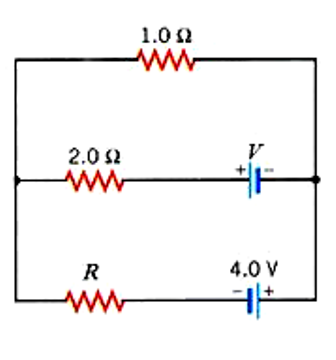# Find the magnitude and direction of the current in the 2.0 ? resistor in the circuit given...

## Question:

Find the magnitude and direction of the current in the 2.0 {eq}\Omega {/eq} resistor in the circuit given below. (Let R = 4.5 {eq}\Omega {/eq} and V = 1.0 V.)## Nodal Analysis:

Nodal analysis or branch current method is used in a parallel network to calculate the potential difference across the respective branches.

It is given as -

{eq}\displaystyle \frac{V_1}{R_1} - \frac{V_2}{R_2} = 0 \implies \text{the sum of incoming currents is equal to the outgoing currents.} {/eq}

Let the direction of current in both loops be anti-clockwise.

{eq}\text{By nodal analysis - }\\ \displaystyle \frac{1 - V}{2} + \frac{-4 - V}{4.5} =...

Become a Study.com member to unlock this answer! Create your account